Next: SUMMARY Up: Crozzle: an NP-Complete Problem Previous: INTRODUCTION

# R-BY-C CROZZLE IS      NP-COMPLETE

To prove that a problem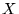is NP-Complete it is sufficient to show that (1)is NP-hard and (2)is in NP . Problemis NP-hard if there is a deterministic polynomial-time reduction from some problem in NP to. Since reductions compose, this implies that every problem in NP can be reduced to. Problemis in NP if all instances ofcan be solved in non-deterministic polynomial time.

Gower and Wilkerson argue that R-by-C Crozzle is NP-hard, but not in NP. They prove R-by-C Crozzle is NP-hard by reducing the exact 3-set cover problem to the R-by-C Crozzle problem. The exact 3-set cover problem is defined as follows: For a set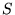and a set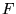, a collection of sets each having three elements from, a solution to the exact 3-set cover problem is a subset ofwhere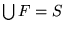and each member ofappears in exactly one element of.

THEOREM 1.  . Exact Cover by 3-sets reduces to R-by-C Crozzle.

Gower and Wilkerson also argue that R-by-C Crozzle is not in NP because

the minimum amount of work required is an examination of each square (i.e., on the order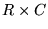). The number of steps is dependent upon the values of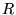and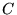rather than the size of the inputs. Since there is no relationship betweenand the number (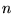) of words in the list, there cannot be a polynomial-time algorithms to check possible solutions for all values ofand, and. Therefore R-by-C Crozzle is not in NP.''

We argue that R-by-C Crozzle is in fact in NP by showing that the number of steps taken to find the highest scoring solution is dependent on the size of the input and not onand. Recall that the words placed on the grid must form an interconnected unit. A bound is found not in the number of words, but in the lengths of the words. Let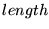be the sum of the lengths of the input words. Neither the width nor the height of the words placed on the grid can exceed. Thus at most a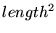portion of thegrid need by considered2. This relationship is used in the following theorem.

THEOREM 2. R-by-C Crozzle is NP-complete.

PROOF. Theorem 1 proves the R-by-C Crozzle is NP-hard. What remains is to prove that R-by-C Crozzle is in NP. One way of doing this is to provide a non-deterministic polynomial time algorithm for solving R-by-C Crozzles. The following algorithms solves an instance of the R-by-C Crozzle problem in non-deterministic polynomial time.

1
Read in,, and the Words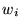.

2
Compute length =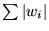.

3
Let R = minimum(R, length) and

C = minimum(C, length).

4
Non-deterministically pick those words that will be used in the solution.

5
Non-deterministically assign each word a starting row, starting column, and orientation (UP-and-DOWN or BACK-and-FORTH).

Steps 1, 2, 4, and 5 take linear time (steps 4 and 5 make a linear number of non-deterministic choices). Step 3 takes constant time.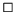Since the original Crozzle problem found in The Australian Women's Weekly is a restricted version of R-by-C Crozzle, we have the following corollary:

COROLLARY. The original Crozzle problem is NP-complete.

Next: SUMMARY Up: Crozzle: an NP-Complete Problem Previous: INTRODUCTION